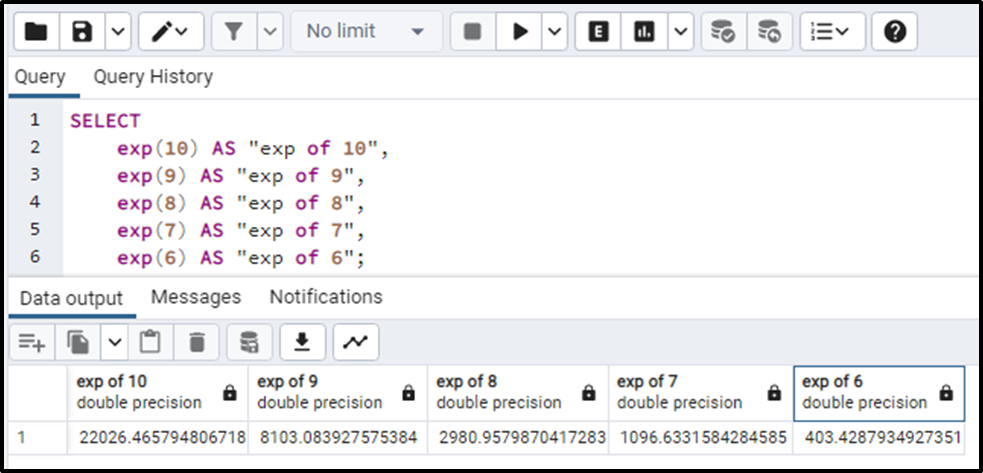##### How the Exp() Function Works in PostgreSQL

In PostgreSQL, the exp() function finds the natural constant "e" raised to the power that is specified. The power is passed into the function as an argument. The number is a numeric value that can be positive, negative, zero, decimal, or an integer. The function will return the exponential value of the number you specify.

## How to Use Exp() Function in PostgreSQL?

The Exp() function in Postgresql takes a numeric value as an input and returns a value having a Numeric data type or specifically DOUBLE PRECISION. The basic syntax for the function is:

`Exp(Numeric_Value);`

The function returns a NULL if the numeric values passed as an argument are NULL. It will throw an error when the value passed is of some other data type than numeric.

Let’s move toward an example that will clearly elaborate on the concept.

Example

The simple query for the Exp() function is given below:

```SELECT
exp(10) AS "exp of 10",
exp(9) AS "exp of 9",
exp(8) AS "exp of 8",
exp(7) AS "exp of 7",
exp(6) AS "exp of 6";```

The output of the given query will be the additional columns having the values of the exp(values) like this:In the above output, we can clearly see that the returned data type of this function is DOUBLE PRECISION. So this was all about the Exp() function.

## Conclusion

The Exp() function finds the exponential value of the specified power. This numeric value needs to be passed into the function. It may contain positive, negative, zero, decimal, or integer values. The function returns the exponentiation of a number that is specified as an argument and that value will have DOUBLE PRECISION data type.